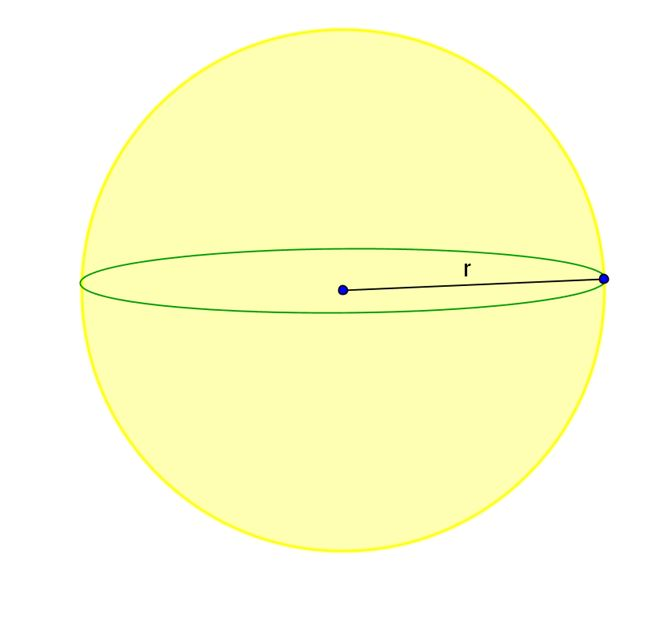# Surface Area and Volume of a Sphere

The surface area of a sphere is the number of square units that will exactly cover its surface.The formula for the surface area of a sphere is given by:

A = 4r²?

where A represents the area and r represents the radius.

If you already know the surface area and need to find the radius, we can rearrange the above formula and solve it for r:

and now take the square root of both sides:

divide both sides with 4?

The volume of a sphere is the number of cubic units that will exactly fill the sphere.

Volume of a sphere is given by:

where V represents the volume and r is the radius.

Again, we can solve the above formula for r and thus find the radius if we are given the volume:

and now take the third root of both sides

divide both sides with

## Interesting fact

The shape with the smallest possible surface area for a given volume is a sphere. Or in other words, the sphere is a shape with the largest volume for a fixed surface area.

The sphere therefore appears in nature in water drops, bubbles, planets etc.

## Example I

Find the surface area and the volume of a sphere with radius 5.

To do this, simply plug in r=5 into the formulas.

For the area:

We can plug in 3.14? for and get:

For the volume:

Again, we can plug in 3.14 for ? and get:

## Example II

Find the radius of a sphere with a volume of 65.42.

Now we’ll use the last formula provided above, the one solved for r. Plug in 65.42 for V and 3.14 for ?:

## More Examples

[box type=”success” align=”” class=”” width=””]You can check out our Geometry video course for more examples on Surface Area and Volume of Spheres. Our instructors will explain each example problem clearly so you can ace your homework.[/box]

SPECIAL OFFER!

SUCCESS8